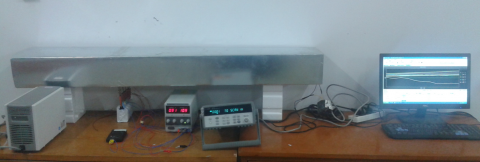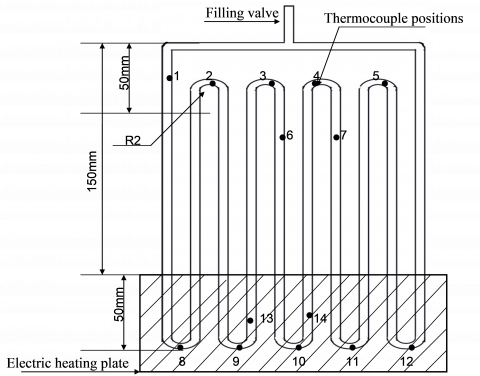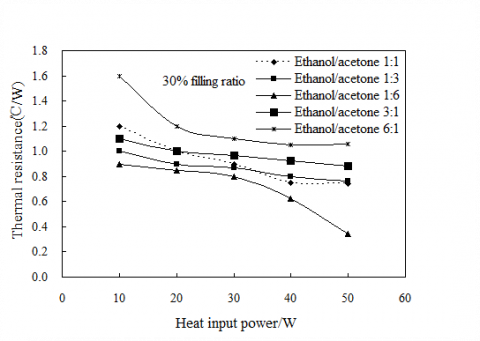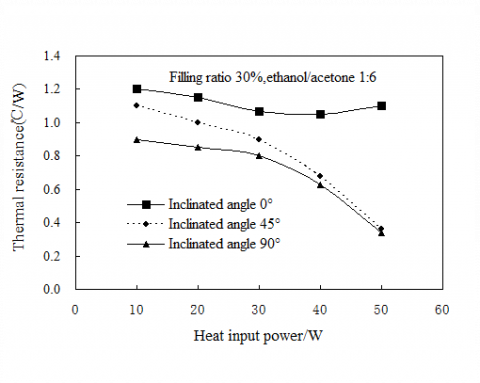# Experimental Study on Thermal Performance of Pulsating Heat Pipe with Ethanol-acetone Mixtures

Experimental Study on Thermal Performance of Pulsating Heat Pipe with Ethanol-acetone Mixtures

Liang CaihangHe Zhuang Yang Yongwang Zeng Si

School of Mechano-Electronic Engineering, Guilin University of Electronic Technology, China, 541004

Corresponding Author Email:
lianghang@guet.edu.cn
Page:
185-190
|
DOI:
http://dx.doi.org/10.18280/ijht.330424
|
Accepted:
|
Published:
31 December 2015
| Citation

OPEN ACCESS

Abstract:

This paper presents experimental results on thermal performance of closed loop pulsating heat pipe (PHP) using copper tube having internal and external diameter with 2.0 mm and 3 mm, respectively. The equal length of evaporator and condenser section was maintained 50 mm, and adiabatic condenser section was maintained 100 mm. Two working fluids, ethanol and acetone, were used with mixing proportion of 6:1, 3:1, 1:1, 1:3 and 1:6, and filling ratios of 30%, 50%, and 70% by volume. The PHP, electrically heated in evaporator and air cooled in condenser with a constant length, was operated at inclined angle of 0°, 45° and 90° and heated power input in a range of 10–50 W. Experimental study on PHP indicated that working fluid was an important factor for the performance of PHP. The result showed that, with the proportion of acetone in the mixing composition increased higher, the thermal performance of PHP became better. The thermal resistance of PHP fell down as the input heating power rose up. It was observed that the performance was best with the volume proportion of ethanol and acetone of 1:6, the filling ratio of 50%, and the inclined angle of 90°.

Keywords:

Pulsating heat pipe, Mixed refrigerant, Thermal performance.

1. Introduction

In the modern society, one of the biggest challenges is the energy problem. Since from the industrial revolution, resources like coal, oil, and natural gas have been almost exhausted. These fossil fuels are non-renewable, so it is very necessary to save energy resources reasonably. LED, being a solid cold light source, is the fourth generation of the new light source just behind of incandescent lamp, fluorescent lamp and high intensity discharge lamp. Compared with the traditional lighting, LED has the advantages of small volume, low power consumption, long service life and good stability [1-2]. As the theme of today is energy saving and emission reduction, semiconductor lighting is becoming a new economic growth point and highly concerned by the academic and industrial circles. However, the LED light efficiency can only reach 10% ~ 20%, about 80% ~ 90% of the energy would convert to heat energy. If the heat energy generated by high power LED chips doesn’t escape timely, the components will accelerate the ageing. Once the junction temperature exceeds the critical temperature, permanent failure of LED may take place [3-5]. Therefore, the heat problem has become an important factor that must be considered in the design of semiconductor lighting system. The most common cooling way for LED at present is the natural convection cooling through the fin heat sinks . The fin heat sinks are made of aluminum, copper or other heat conductive metal. The fin heat sinks are made of aluminum, copper or other heat conductive metal. With the increase requirement of the LED power, the heat exchange area must increase also. The added area leads to increase of not only size and weight but also cost of manufacture [7-9]. Consequently, the traditional method can not solve the heat dissipation problem of high power LED absolutely.

Pulsating Heat Pipe (PHP) was proposed by Japanese scholar H. Akachi in the late of 1990 . The PHP was made of bent capillary which is vacuum pumped and filled with working fluids. It has the advantages of simple structure, low cost, high heat flux heat transfer characteristic and easy to be miniaturized [11-14]. The merits of PHP make it a great potential application and development in the cooling of electronic components with high heat flux. As the internal phase change process of PHP is very complex, non-appropriate theoretical model which can describe the internal movement accurately has already been put forward so far [15-20]. Currently, scholars research the heat transfer performance of pulsating heat pipe by means of experiments to explore the influence of working fluid, filling rate, inner diameter, inclination angle, heat input power, etc [21-22].

Working medium filled inside of the PHP is general a single fluid, which has limitations in application for the characteristics of latent heat, sensible heat, dynamic viscosity and surface tension. Application of mixed refrigerant can combine both sensible heat and latent heat and reduce the viscosity and surface tension of a single fluid. It makes the pulsating heat pipe start up and transfer heat well [24-25]. Therefore, researches on mixed refrigerant PHP will be a trend in the now and future. This paper mainly studies the application of mixtures of ethanol and acetone in a pulsating heat pipe. In the study, the effects of some important parameters (filling ratio, mixing proportion, heating power input, and inclined angle) on the thermal performance of PHP were experimentally investigated.

2. Experimental Setup

2.1 Experimental setup

As shows in Figure  1, the experimental devices consisted of a PHP, electric heating plate, air cooling system, thermocouples and an Agilent data acquisition device. The PHP, indicated in Figure  2, was made up of a copper tube welded into five turns with external diameter of 3 mm and inner diameter of 2 mm. The equal length of evaporator and condenser section was maintained 50 mm, the length of adiabatic section was 100mm.Two types of working fluids, ethanol and acetone, were investigated at different mixing proportion of 6:1, 3:1, 1:1, 1:3 and 1:6, in different filling ratios of 30%, 50%, and 70% by volume. A Eyela DTC-41 vacuum pump was used to vacuum and fill in working fluid inside of the PHP. Before charging another working fluid, the PHP would be purged. The evaporator section of PHP was heated by electric heating plate with the power input in a range of 0–50 W.The heating power was obtained by measuring the electric current and voltage. The condenser section was cooled by forced air in a wind duct. An axial fan was installed at the outlet of a rectangular air duct (1800mm(L) *200mm(W) *150mm(H)).

2.2 Uncertainty analysis

Temperature difference between the evaporator and condenser is widely applied to evaluate the thermal performance of a PHP, and a lower value of thermal resistance usually means higher thermal performance. The overall thermal resistance of a PHP was defined as

$R=\frac{T_{\mathrm{e}}-T_{\mathrm{c}}}{Q}$     (1)

The parameter $T_{e}$ and $T_{c}$ were the average wall temperatures of the evaporator and condenser respectively.

$T_{\mathrm{e}}=\frac{1}{5} \sum_{\mathrm{i}=8}^{12} T_{\mathrm{i}}$     (2)

$T_{\mathrm{c}}=\frac{1}{5} \sum_{\mathrm{i}=1}^{5} T_{\mathrm{i}}$    (3)

Where $Q$ was the heating power input applied to the evaporator can be calculated as

$Q=UI$    (4)

Standard uncertainties can be expressed:

$\frac{\delta Q}{Q}=\sqrt{\left(\frac{d U}{U}\right)^{2}+\left(\frac{d I}{I}\right)^{2}}$     (5)

$\frac{\delta R}{R}=\sqrt{\left(\frac{\delta T_{\mathrm{e}}}{T_{\mathrm{e}}-T_{\mathrm{c}}}\right)^{2}+\left(\frac{\delta T_{\mathrm{c}}}{T_{\mathrm{e}}-T_{\mathrm{c}}}\right)^{2}+\left(\frac{\delta Q}{Q}\right)^{2}}$     (6)

## 1a.png(a) Schematic of experiment setup

## 1b.png(b) Experimental apparatus of PHP

Figure 1. Experimental setup of PHP

## 2.pngFigure 2. Locations of the thermocouples in PHP

The voltage and the current of the power supply were both accurate to 0.5. At 10 W, the ranges of the voltage and current were 0–30 V and 0–5 A, respectively. Under these conditions, the expected uncertainty can be calculated: $U$= 10.9 V, $I$= 0.92 A, $\left(T_{\mathrm{e}}-T_{\mathrm{c}}\right)_{\min }$ = 7.1 ℃ (for ethanol-acetone, mixing ratios of 13:1, filling ratio of 70%). The above equations can be calculated:

$\frac{\delta Q}{Q}=\sqrt{\left(\frac{0.5 \% \times 30}{10.9}\right)^{2}+\left(\frac{0.5 \% \times 5}{0.92}\right)^{2}}=3.05 \%$

$\frac{\delta R}{R}=\sqrt{2 \times\left(\frac{0.1+0.0256}{7.1}\right)^{2}+(3.05 \%)^{2}}=3.94 \%$

The expected maximum uncertainty $U_{\max }$ was

$U_{\max }=2 \max \left\{\frac{\delta Q}{Q}, \frac{\delta R}{R}\right\}=7.88 \%$

By checking the uncertainty for each heat input and working fluid, this was the maximum uncertainty for a small heating power and a low temperature difference.

3. Results and Discussion

3.1 Effect of mixing proportion

The thermal resistance of pulsating heat pipe with different filling ratios (30%, 50% and 70% ) and mixing proportions (1:1, 1:3, 1:6, 3:1, 6:1) were shown in Figure 3.The pulsating heat pipe was operated with the heat input power from 10W to 50W. It could be found that, for working fluids of PHP with different mixing proportions, thermal resistance decreased smoothly with the increasing heat input power. When the filling ratios were 30%, 50% and 70%, the PHP with mixing

proportion of ethanol and acetone 1:6 showed better heat transfer performance than the PHP with other mixing proportions. What’s more, the thermal resistance of pulsating heat pipe was the maximum with mixing proportion of 6:1 when the filling ratio was 30%. It turned to be that, the thermal resistance of PHP would be lower if the occupies of acetone in the mixing proportion got higher.

It was clear that, the performance of working fluid was mainly influenced by the thermo-physical characteristics. Besides, the latent heat of vaporization was the main characteristic that strongly affected the heat transfer performance of PHP. The boiling points and the latent heat of ethanol were larger than acetone. In addition, the dynamic viscosity of ethanol was significantly higher than that of acetone and the surface tension of ethanol and acetone was basically the same. To operate inside of the PHP, the working medium must overcome the friction, the surface tension and viscosity etc. Therefore, if the possession of ethanol in the mixing proportion got higher, it would be more difficult to overcome the dynamic viscosity for operation in the pulsating heat pipe.

## 3a.png(a) 30% filling ratio

## 3b.png(b) 50% filling ratio

## 3c.png(c) 70% filling ratio

Figure 3. Thermal resistance of PHP with different filling ratios and mixing proportions

3.2 Effect of filling ratios

Compared with the Figure 3 (a) and (c), the thermal resistance of PHP in filling ratio 30% with heat input range from 10W to 50W was the maximum when the mixing proportion of ethanol and acetone was 6:1. While the mixing proportion of the maximum thermal resistance turned to be 3:1 when the filling ratio was 70% and heat input range was from 10W to 40W. It was observed that the thermal resistance of PHP with mixing proportion of 1:1, 1:3 and 6:1 appeared to be almost the same with heat input from 20W to 30W in filling ratio 70%.

It was indicated that the filling ratio impacted the oscillation of working fluid in the PHP obviously. For the PHP with lower filling ratio, it was more easily for the working fluid to evaporate in the evaporation section. The PHP with lower filling ratio would show better heat transfer performance if the heat flux was very low. Nevertheless, the heat transfer capability of the PHP was also limited to the small amount of the liquid working fluid. Consequently, the entire influence of the filling ratio on the thermal performance of PHP was based on the interaction between the driving force and the heat transfer capability of the working fluid.

3.3 Effect of inclined angle

## 4.pngFigure 4. Thermal resistance of PHP in different inclined angle

From the Figure 3, it was observed that the heat transfer performance of PHP with mixing proportion 1:6 was much better than others from input power 10W to 50W. Moreover, PHP with lower filling ratio could start up easily. Therefore, pulsating heat pipe in filling ratio 30% and mixing proportion 1:6 was investigated in three cases (0 degrees, 45 degrees and 90 degrees), to find out how the inclined angle effect on the heat transfer performance of PHP. The heat pipe was arranged horizontally with inclined angle of 0 degrees and vertically with inclined angle of 90 degrees. From the figure 4 we could see that, thermal resistance of PHP with inclined angle of 0 degrees was obviously higher than that of 45 degrees and 90 degrees. With the increase of heat power, thermal resistance of PHP with inclined angle of 45 degrees and 90 degrees had a steady decline. While, thermal resistance of 0 degrees descended slowly during the input power of 10~40W and then ascended exceeding the heat power of 40W. As a result, heat transfer performance of pulsating heat pipe was the best when the inclination angle is 90 degrees.

4. Conclusions

In this paper, the effects of mixing proportion, heating power input, filling ratio by volume, and inclined angle on the thermal performance of PHP were experimentally investigated. Experimental study on PHP indicated that mixing proportion of working fluid was an important factor for the performance of PHP. The results showed that, with the proportion of acetone in the mixing composition getting higher, the thermal performance of PHP becoming better. The performance was the best when the volume proportion of ethanol and acetone was 1:6. High heat flux in evaporator section could enhance the oscillation of working fluid in the pipe. At the range of 10W to 50W, the thermal resistance of PHP fell down as the input heating power rose up. What was more, the filling ratio impacted the thermal performance of PHP obviously. The optimal filling ratio in this paper was 50%. Finally, the appropriate inclined angle of PHP was 90°.

Acknowledgments

This project is jointly supported by National Natural Science Foundation of China (No. 51106032), and by Guangxi Key Lab of Manufacturing System & Advance Manufacturing Technology Foundation(No. 13-051-09-008Z), and by Guangxi Experiment Center of Information Science Foundation(No. 20130315) Innovation Project of graduate student Funded by Guilin University of Electronic Technology(No. GDYCSZ201402).

Nomenclature
 $Q$ electric heating capacity/heat input, W $R$ thermal resistance of the PHP, ℃/W $T_{e}$ temperature of the condensation section, ℃ $T_{c}$ temperature of the condensation section, ℃ $T_{i}$ average temperature of the thermocouple at steady state, ℃ $U_{\max }$ expected maximum uncertainty
References

1. M. Alan, “Solid state lighting-a world of expanding opportunities at LED 2002,” III–V Review, vol. 16, pp. 30-33, 2003.

2. M. Alan, “LED 2005 illuminates,” III–V Review, vol. 18, pp. 30-35, 2005.

3. N. Narendran, Y.M. Gu, “Life of LED-based white light sources,” Journal of Display Technology, vol. 1, pp. 167-171, 2013. DOI: 10.1109/JDT.2005.852510.

4. E. Nogueira, M. Vazquez, J. Mateos, “Accelerated life test of high luminosity AlGaInP LEDs,”Microelectronics and Reliability, vol. 52, pp. 1853-1858, 2012. DOI: 10.1016/j.microrel.2012.06.125.

5. A. Mehmet, P. James, W. Stanton, “Thermal challenges in the future generation solid state lighting application,”Proc. 2002 Intersociety Conference on Thermal and Thermomechanical Phenomena in Electronic Systems (ITherm 2002), San Diego, California, USA, 2002.

6. C. Alvin, W. Chu, C.H. Chen, “Thermal analysis of extruded aluminum fin heat sink for LED cooling application,” Assembly and Circuits Technology Conference (IMPACT), 2011. DOI: 10.1109/IMPACT.2011.6117207.

7. M. R. Shaeri, M. Yaghoubi, “Thermal enhancement from heat sinks by using perforated fins,” Energy Conversion and Management, vol. 50, pp. 1264－1270, 2009. DOI: 10.1016/j.enconman.2009.01.021.

8. C.T. Chen, C.K. Wu, C. Hwang, “Optimal design and control of CPU heat sink processes,” IEEE Trans. Components and Packaging Technologies, vol. 31, pp. 184-195, 2008.

9. S. Ndao, S. Peles, M.K. Jensen, “Multi-objective thermal design optimization and comparative analysis of electronics cooling technologies,” International Journal of Heat Mass Transfer, vol. 52, pp. 4317–4326, 2009. DOI: 10.1016/j.ijheatmasstransfer.2009.03.069.

10. H. Akachi, Structure of a Heat Pipe, US: 4921041, 1990-05-01.

11. Q.J. Cai, C.L. Chen, J.F. Asfia, “Operating characteristic investigations in pulsating heat pipe,” Journal of Heat Transfer, vol. 128, pp. 1329–1334, 2006. DOI: 10.1115/1.2349509.

12. Y.W. Zhang, A. Faghri, “Advances and unsolved issues in pulsating heat pipes,” Heat Transfer Engineering, vol. 29, pp. 20–44, 2008. DOI: 10.1080/01457630701677114.

13. L. Xiao, Y.D. Cao, “Recent advances in pulsating heat pipes and its derivatives,” Journal of Enhanced Heat Transfer, vol. 19, pp. 213–31, 2012. DOI: 10.1615/JEnhHeatTransf.2012001896.

14. A. Faghri, “Review and advances in heat pipe science and technology,” Journal of Heat Transfer, vol. 134, pp. 1-18, 2012. DOI: 10.1115/1.4007407.

15. Y.W. Zhang, F. Amir, “Heat transfer in a pulsating heat pipe with open end,” International Journal of Heat and Mass Transfer, vol. 45, pp. 755-764, 2002. DOI: 10.1016/S0017-9310(01)00203-4.

16. H.H. Yang, S. Khandekar, M. Groll, “Simulation study of flow patterns in single closed-loop pulsating heat pipe,” Fluid Machinery, vol. 35, pp. 58-69, 2007.

17. D.Z. Yuan, W. Qu, T.Z. Ma, “Flow and heat transfer of liquid plug and neighboring vapor slugs in a pulsating heat pipe,” International Journal of Heat and Mass Transfer, vol. 53, pp. 1260–1268, 2010. DOI: 10.1016/j.ijheatmasstransfer.2009.12.042.

18. S. Khandekar, P. Charoensawan, M. Groll, “Closed loop pulsating heat pipes Part B: visualization and semiempirical modeling,” Applied Thermal Engineering, vol. 23, pp. 2021-2033, 2003. DOI: 10.1016/S1359-4311(03)00168-6.

19. H. Yapici, N. Kayatas, “Study on transient local entropy generation in pulsating fully developed laminar flow through an externally heated pipe,” Heat Mass Transfer, vol. 43, pp. 17-35, 2006.

20. S. Khandekar, S. Manyan, M. Groll, et al, “Two phase flow modeling in closed loop pulsating heat pipes,” Proceedings of the 13th International Heat Pipe Conference, Shanghai, China, pp. 275-282, 2004.

21. P. Charoensawan, S. Khandekar, M.Groll, et al, “Closed loop pulsating heat pipes Part A: parametric experimental investigations,” Thermal Engineering, vol. 23, pp. 2009-2020, 2003. DOI: 10.1016/S1359-4311(03)00159-5.

22. S. Khandekar, A.P. Gautam, P.K. Sharma, “Multiple quasi-steady states in a closed loop pulsating heat pipe,”International Journal of Thermal Sciences, vol. 48, pp. 535-546, 2009. DOI: 10.1016/j.ijthermalsci.2008.04.004.

23. S. Khandekar, N. Dollinger, M. Groll, “Understanding operational regimes of closed loop pulsating heat pipes: an experimental study,” Applied Thermal Engineering, vol. 23, pp. 707-719, 2003. DOI: 10.1016/S1359-4311(02)00237-5.

24. Y. Zhu, X.Y. Cui, H. Han, S.D. Sun, “The study on the difference of the start-up and heat-transfer performance of the pulsating heat pipe with water-acetone mixtures,” International Journal of Heat and Mass Transfer, vol. 77, pp. 834-842, 2014. DOI: 10.1016/j.ijheatmasstransfer.2014.05.042.

25. P.R. Pachgharea, A.M. Mahalleb, “Effect of pure and binary fluids on closed loop pulsating heat pipe thermal performance,” Chemical Civil and Mechanical Engineering Tracks of 3rd Nirma University International Conference, Procedia Engineering, pp. 624–629, 2013. DOI: 10.1016/j.proeng.2013.01.088.